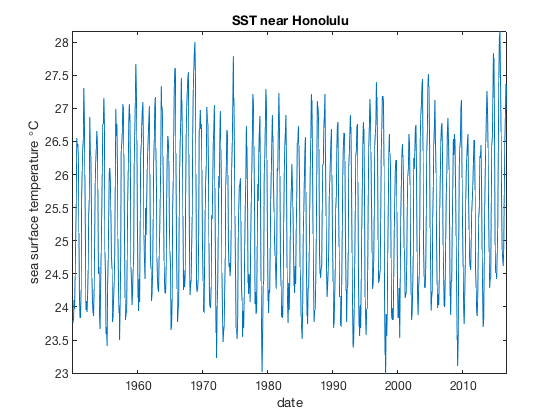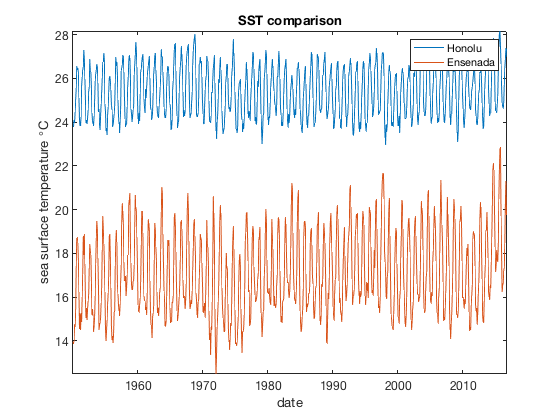# near2 documentation

near2 finds the subscript indices of the point in a grid closest to a specified location.

## Syntax

```[row,col] = near2(X,Y,xi,yi)
[row,col,dst] = near2(...)```

## Description

[row,col] = near2(X,Y,xi,yi) returns the row and column corresponding to the X,Y grid point with the shortest euclidean distance to the point xi,yi.If xi or yi is equidistant between two X or Y grid points, only the first of the two equally valid rows or columns is returned.

[row,col] = near2(X,Y,xi,yi,mask) ignores X,Y grid points corresponding to false mask values. This option may be useful near data boundaries, where the nearest point in the data grid could correspond to NaN data in the time series.

[row,col,dst] = near2(...) also returns the euclidean distance dst from the point (X(row,col),Y(row,col)) to the point (xi,yi).

## Example 1

Consider this grid of X and Y values, and a special location of interest xi,yi, which we'll mark with a red x:

```[X,Y] = meshgrid(50:5:300,480:5:675);

xi = 103.2;
yi = 517;

plot(X,Y,'.','color',rgb('light blue'))

hold on
plot(xi,yi,'rx')
axis equal tight

xlabel 'x value'
ylabel 'y value'
```Use near2 to determine the subscript indices of the X,Y grid points closest to xi,yi and mark that grid point with a blue circle:

```[row,col] = near2(X,Y,xi,yi);

plot(X(row,col),Y(row,col),'bo')
```If you also want to know the distance from (xi,yi) to (X(row,col),Y(row,col)), request the third function output:

```[row,col,dst] = near2(X,Y,xi,yi);
dst
```
```dst =
2.6907
```

...and we see that our point of interest is about 2.69 units away.

## Example 2: Climate data application

Suppose you want a time series of sea surface temperatures near Honolulu, Hawaii (21.3 N, 157.8 W). You could do it with geomask and local or you could use near2 to find the row and column of the SST grid cell closest to Honolulu. Here's the situation:

```load pacific_sst

[Lon,Lat] = meshgrid(lon,lat);

figure
imagescn(lon,lat,mean(sst,3))
cmocean thermal
hold on
plot(-157.8,21.3,'bo')
```The SST grid cell closest to Honolulu can be found like this:

```[row,col] = near2(Lat,Lon,21.3,-157.8)
```
```row =
20
col =
12
```

With row and col, the geographic coordinates of the grid cell are then given by

```[Lat(row,col) Lon(row,col)]
```
```ans =
21.5000 -157.5000
```

The SST time series at the grid point given by row,col is then easy to plot. The only trick is you'll need to use the squeeze command to squeeze the 1x1x802 time series into an 802x1 array so the plot function will understand it:

```sst_honolulu = squeeze(sst(row,col,:));

figure(22) % giving this figure a number so we can come back to it later.
plot(t,sst_honolulu)
axis tight
datetick('x','keeplimits')
xlabel 'date'
ylabel 'sea surface temperature \circC'
title 'SST near Honolulu'
```## Example 3: Masking NaNs

Sometimes the location of interest sits close to a boundary, such that you try to get the time series at the grid cell closest to your location of interest, but all you get are NaNs in return. For example, you might be interested in sea surfaces temperatures close to a city, but because most cities are on land, and most sea surface temperatures are found at sea, there's a good chance that the time series of SSTs closest to your favorite city is just a bunch of NaNs.

For example, consider this point near Ensenada, Mexico:

```figure(33) % giving this figure a number so we can come back to it later.
imagescn(lon,lat,mean(sst,3))
cmocean thermal
hold on
borders('countries','color',rgb('gray'))
plot(-116,31,'rp')
'horiz','center','vert','top')
axis([-133 -94 10 55])

% Get nearest grid indices and label them:
[row,col] = near2(Lat,Lon,31,-116);
plot(Lon(row,col),Lat(row,col),'bs')
text(Lon(row,col),Lat(row,col),' nearest grid cell',...
'color','b','vert','middle')
```And sure enough, all the SST data in the grid cell closest to Ensenada are NaNs:

```% Get 1D time series of SSTs near Enenada:

% How many Enesnada SSTs are finite?
```
```ans =
0
```

To find the closest SST grid cell where data are not NaNs, start by defining a mask of good grid cells, like this:

```% Determine which grid cells have finite data:

figure
title 'this is the mask'
```The mask above shows good (true) grid cells in yellow, and bad (false) grid cells in blue. We can now enter the mask into near2, to find which good grid cells are closest to Ensenada:

```[row,col] = near2(Lat,Lon,31,-116,mask);

figure(33) % goes back to the map
plot(Lon(row,col),Lat(row,col),'ks')
text(Lon(row,col),Lat(row,col),'nearest finite grid cell ',...
'horiz','right','vert','middle')
```The time series of finite SSTs close to Ensenada can now be compared to the Honolulu SSTs like this:

```sst_ensenada = squeeze(sst(row,col,:));

figure(22) % goes back to the Hawaii figure
hold on
title 'SST comparison'
```## A note the order of georeferenced grids

It's usually quite important to keep track of latitudes and longitudes separately. Some grids are built like

`[Lat,Lon] = meshgrid(lat,lon);`

whereas other grids are built like

`[Lon,Lat] = meshgrid(lon,lat);`

and plotting coordinates by

`plot(lon,lat)`

will not produce the same results as

`plot(lat,lon)`

In contrast, near2 will always produce the same results whether you treat the longitudes as x or y values. That is, both of the following syntaxes will produce the correct row and column of the SST grid cell closest to Honolulu:

```[row,col] = near2(Lat,Lon,21.3,-157.8)

[row,col] = near2(Lon,Lat,-157.8,21.3)
```
```row =
20
col =
12
row =
20
col =
12
```

The only caveat here is that with georeferenced grids, you probably don't want to use the optional third output dst. That's because the Euclidean distance in degrees latitude and longitude does not make any sense, given that degrees longitude do not represent the same distance as degrees latitude.

## Author Info

This function is part of the Climate Data Toolbox for Matlab. The function and supporting documentation were written by Chad A. Greene of the University of Texas at Austin.